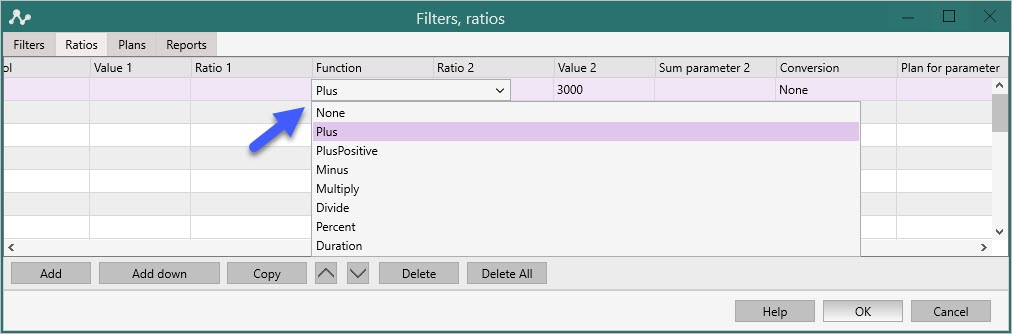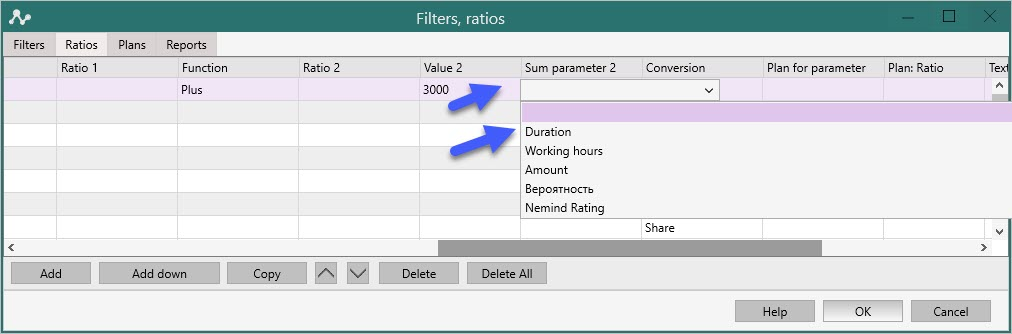To properly display this page you need a browser with JavaScript support.×
 Ratios
Ratios

Ratios is a key concept in Nemind analytics. Ratios are mathematical metrics that have a name and calculation settings. For example, the ratio of the Deal report “Created” calculates the count of created deals. This ratio is displayed in the Kanban, Ratios and Cross tables, it can be selected for all charts, it is displayed in tooltips. It will be automatically applied for all aspects - by employees, sources, and so on. If you create a new ratio, it will also appear in all places in the program that work with ratios.Often create an ratio "Measurements" (Presentations) - which shows the count of measurements. For example, “measurement” is determined from the custom field of the deal “Measurement date” - then in the ratio it is enough to select the date parameter “Measurement date”. This is enough to calculate measurements for employees, taking into account the selected period. The "Measurements" ratio will automatically appear in tables and diagrams. You can always edit its name and calculation method.

Another common example is a special sum, such as deals costs, that are also entered in a custom field. You can make the “Costs” ratio - literally in two clicks, and for the ratio with the sum, choose the average, maximum or minimum. There are standard options for customizing conversions, stakes, and plans. You can create ratios that use other ratios — for example, the Conversion to Meter ratio will use the Metered ratio and take a percentage of another Target Leads ratio.

Ratios settings
At this point, we will consider the meaning of each column in the "Ratio" tab of the "Filters and Ratios" functionality.
An example of use is shown here.

Let's create a new ratio. By default, the parameters of the ratio will be set as follows:1. The column "Name" contains the name of the Ratio, it can be edited (if the ratio is not already used in other ratios):2. The "Filter" column contains a list of all created filters from the "Filters" tab:The column "Type" contains a list of ratios: Count, Sum, Average, Maximum and Minimum value.
Count is the number of lines in the "Data" tab, Sum is configured in the Project Settings.The "Period" column contains the period for which the Ratio is calculated.
Note: in the latest versions, for all new ratios, it automatically shows the change in the current to the previous period, so this setting is rarely used.The periods are set in the settings of the "Main" tab of the main menu of the program:The column "Sum parameter" contains Aspects in numerical values ​​for calculation. The default sum parameter is configured in the Project Settings. Any other parameter for Sum can be applied here:The column "Date Parameter" is configured by default in the Project Settings. Any other parameter for Date can be applied here:Uniqueness - selects unique values ​​from a common database by a specific parameter, which can be selected from the drop-down menu.In the "Symbol" Column, enter the Symbol you need for the ratio (%, \$, etc.)

The block of mathematical calculations consists of 5 columns.Columns "Value 1" "Value2" - here you can put any numerical value with which you need to perform mathematical operations:See the result:Columns "Ratio1" and "Ratio2" - select the existing ratios with which you want to perform a mathematical action.Column "Function" - select the required mathematical action:Value1 and Ratio1 are on the left side of the equation, Value2 and Ratio2 are on the right side of the equation. The "function" is located between them, i.e. simplest formula:

x + y = z

in the program it will look like this:

Value1 Plus Ratio2 = New Ratio, or
Value 1 Plus Value2 = New Ratio1, or
Ratio1 Plus Value 2 = New Ratio2, or
Ratio1 Plus Ratio2 = New Ratio3, where

x - either Value1 or Ratio1
y - either Value2 or Ratio 2
+ - A function that can be changed to any other.
z is a new ratio, the name of which is entered at the very beginning.

You will see the final calculation in the "Ratios" tab when you exit this menu.

Column "Sum parameter 2" - allows you to change the parameter for the Ratio2 Sum value in the previous formulaColumn "Conversion" - will show the share or distribution of the ratio to the total sum, similar to the "Conversion" functionality (previous Bars), where:
- share is the share of the ratio in each of the segments;
- distribution is the distribution of ratios between segments within each sectionColumns for Plan -  assigns plans from the "Plan" tab to a specific Section, for example, to the "Created" or "Responsible" section. Calculated here.Text ratio allows you to enter the value of text columns from Data inside the Table.Column for working with ScoringAn Ratio that allows you to move an Ratio from another open report to thisThe button enables the If functionality, where you can find more precise conditions for this ratio